Next: Equations of Incompressible Fluid Up: Mathematical Models of Fluid Previous: Navier-Stokes Equation

# Energy Conservation

Consider a fixed volumesurrounded by a surface. The total energy content of the fluid contained withinis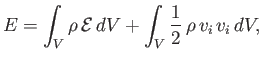(1.59)

where the first and second terms on the right-hand side are the net internal and kinetic energies, respectively. Here,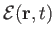is the internal (i.e., thermal) energy per unit mass of the fluid. The energy flux across, and out of, is [cf., Equation (1.29)]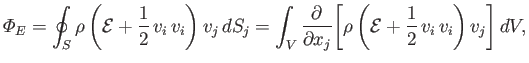(1.60)

where use has been made of the tensor divergence theorem. According to the first law of thermodynamics, the rate of increase of the energy contained within, plus the net energy flux out of, is equal to the net rate of work done on the fluid within, minus the net heat flux out of: that is,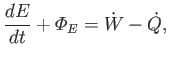(1.61)

where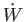is the net rate of work, and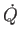the net heat flux. It can be seen that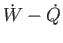is the effective energy generation rate within[cf., Equation (1.31)].

The net rate at which volume and surface forces do work on the fluid withinis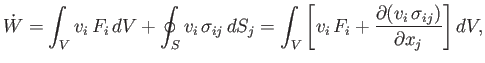(1.62)

where use has been made of the tensor divergence theorem.

Generally speaking, heat flow in fluids is driven by temperature gradients. Let thebe the Cartesian components of the heat flux density at position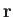and time. It follows that the heat flux across a surface element, located at point, is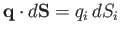. Let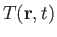be the temperature of the fluid at positionand time. Thus, a general temperature gradient takes the form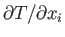. Let us assume that there is a linear relationship between the components of the local heat flux density and the local temperature gradient: that is,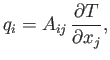(1.63)

where theare the components of a second-rank tensor (which can be functions of position and time). In an isotropic fluid we would expectto be an isotropic tensor. (See Section B.5.) However, the most general second-order isotropic tensor is simply a multiple of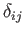. Hence, we can write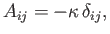(1.64)

where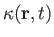is termed the thermal conductivity of the fluid. It follows that the most general expression for the heat flux density in an isotropic fluid is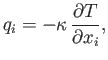(1.65)

or, equivalently,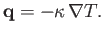(1.66)

Moreover, it is a matter of experience that heat flows down temperature gradients: that is,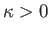. We conclude that the net heat flux out of volumeis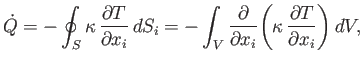(1.67)

where use has been made of the tensor divergence theorem.

Equations (1.59)-(1.62) and (1.67) can be combined to give the following energy conservation equation: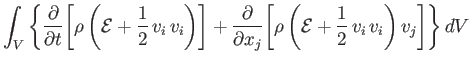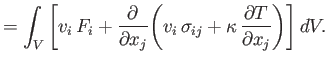(1.68)

However, this result is valid irrespective of the size, shape, or location of volume, which is only possible if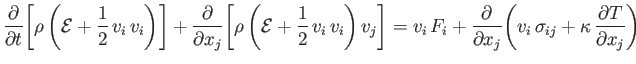(1.69)

everywhere inside the fluid. Expanding some of the derivatives, and rearranging, we obtain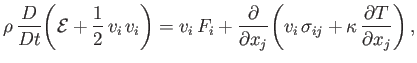(1.70)

where use has been made of the continuity equation, (1.40). The scalar product of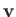with the fluid equation of motion, (1.53), yields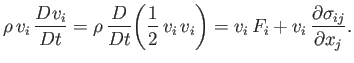(1.71)

Combining the previous two equations, we get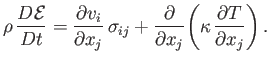(1.72)

Finally, making use of Equation (1.26), we deduce that the energy conservation equation for an isotropic Newtonian fluid takes the general form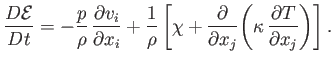(1.73)

Here,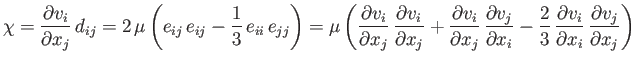(1.74)

is the rate of heat generation per unit volume due to viscosity. When written in vector form, Equation (1.73) becomes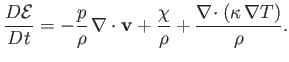(1.75)

According to the previous equation, the internal energy per unit mass of a co-moving fluid element evolves in time as a consequence of work done on the element by pressure as its volume changes, viscous heat generation due to flow shear, and heat conduction.Next: Equations of Incompressible Fluid Up: Mathematical Models of Fluid Previous: Navier-Stokes Equation
Richard Fitzpatrick 2016-03-31